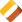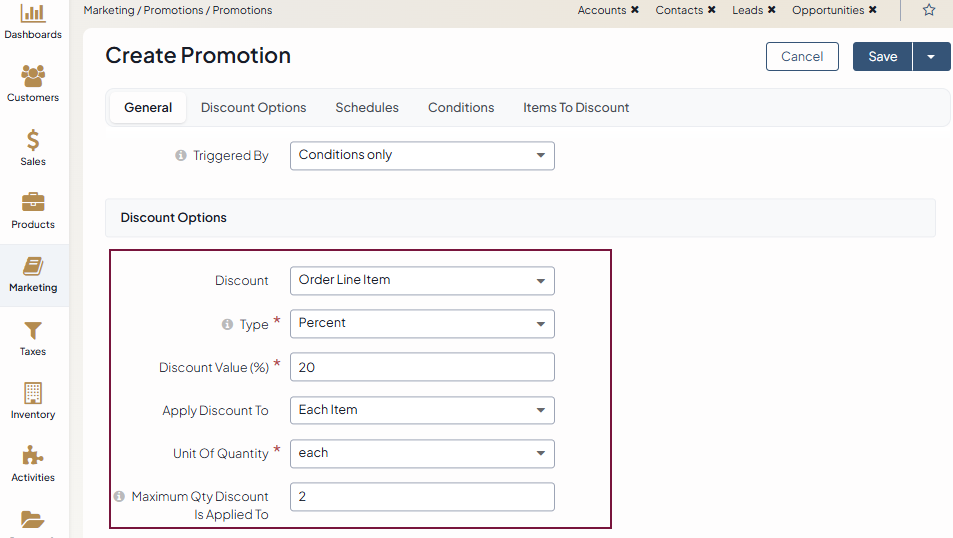# Calculate Order Total in Promotions¶

We are going to illustrate how price calculations work in Oro using the example below, where the rounding precision is set to 2:

Product Price Quantity
TAG3 \$1.0149 3
TAG2 \$3.0209 1
1. Row Totals

Row totals are calculated for each line item, then summarized and rounded for the subtotal amount.

The calculated subtotal is:

```round (1.0149*3 + 3.0209*1) = round (6.0656) = 6.07\$.
```
1. Order Discounts

When order discounts (i.e Special Discounts) are used, the line item subtotal calculated previously is used.

For example, if we add a 10% order discount (on the order edit page in the back-office), the calculated discount subtotal is:

```round (6.07/10) = round (0.607) = 0.61\$
```
2. Promotion Discounts

If you use promotion discounts, their calculation may be different depending on the promotion discount type.

The following is an example of a complex promotion with the Order Line Item discount:

• Type = Percent
• Discount Value = 20%
• Apply Discount to = Each Item
• Max Quantity = 2
• For TAG3In this case we take the price for one item, multiple by the percent value and the maximum quantity, and then summarize all discounts from promotions, and round the subtotal. The calculated discount subtotal is:

```round((1.0149-10%) * 0.2 * 2 (maximum quantity in 3 existing)) = round(0.365364) = -0.37\$
```
3. Shipping Cost

Flat Rate Shipping Cost + \$10.00

1. Taxes

10% sales tax +0.61\$ (round((1.0149*3 + 3.0209*1) * 10%) = round(0.60656) = 0.61\$)

2. Grand Total

```6.07 - 0.61 - 0.37 + 10 + 0.61 = 15.70\$.
```